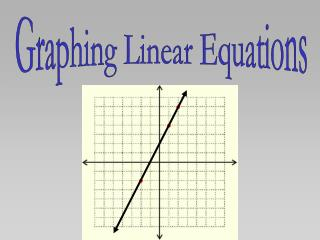DownloadDownload PresentationGraphing Linear Equations

# Graphing Linear Equations

Télécharger la présentation## Graphing Linear Equations

- - - - - - - - - - - - - - - - - - - - - - - - - - - E N D - - - - - - - - - - - - - - - - - - - - - - - - - - -
##### Presentation Transcript

1. Graphing Linear Equations

2. Linear Equation: an equation whose graph forms a line. Linear means of a line. So a linear equation is the equation of a line. In linear equations, all variables are taken to the first power. is linear. is not. Why?

3. Suppose you were asked to graph the solution set of the linear equation, You could start by creating a table of values.

4. Choose any number to use as x or y . . .

5. . . . and substitute the number for the variable. Then calculate to find,

6. Continue, until you have 3 sets of x and y values.

7. Continue, until you have 3 sets of x and y values.

8. With your table completed for three sets of values, you are ready to graph the line.

9. When x is 0, y is 3, so plot the point, (0, 3).

10. And when x is 3, y is 0, so plot the point, (3, 0).

11. If they do line up, you just need to connect the dots. If the three points do not line up, you need to find your mistake.

12. This is the graph of the linear equation,

13. Create a table of values for each equation, and graph it on grid paper: 1. 2. 3. 4.